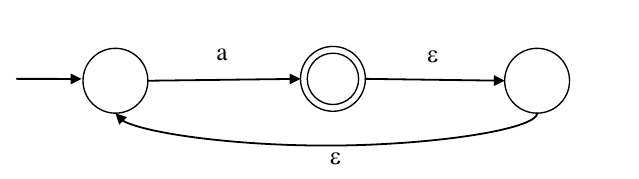+33 votes
4.1k views

What is the complement of the language accepted by the NFA shown below?
Assume $\Sigma = \{a\}$ and $\epsilon$ is the empty string.1. $\phi$
2. $\{\epsilon\}$
3. $a^*$
4. $\{a , \epsilon\}$

asked
edited | 4.1k views
0
What will be the NFA for the complement of the lang?

All state transition arrows will be reversed and non-final states will be final and vice-versa. Is it correct?
+1
arrows will not be reversed.....
0

Assume $Σ={a}$ and $ϵ$ is the empty string.$L=\left\{a^{+}\right\}$

$(1)$ What is the complement of the language accepted by the $NFA?$

$\overline{L}=\left\{ϵ\right\}$
$(2)$ What is the language which accepts complement of $NFA?$$L_{1}=\left\{ϵ,(a+ϵ)^{+}\right\}$

Please correct me if i'm wrong$?$

+2
No,  it is not correct as it is epsilon-nfa. In nfa,   we do not get a complement by complementing the state diagram. You have to complement the language.  So,  language accepted here is L={a+}.

So,  it's complement would be L'={€}
0
which one is not correct??

## 7 Answers

+50 votes
Best answer
The language being accepted is $a^+$. So, complement of the language is $\{\epsilon\}$.
answered by Veteran (400k points)
selected by
+2
How will aa be produced here ?  after one a it goes to final state . What hapens on reading the 2nd 'a'  ?
+16
@gokou from final state using epsilon we can again come back to start state..and the process repeats!!
0
Is this true?: If L = $\phi$ then L* = $\{\epsilon\}$
0
@habed yes true
0
Sir, I have the same query. If in complementation, arrows are not reversed, then there are always epsilon paths from the non-final state(middle state) to the final states. So language accepted should be a*.

But again, your solution also seems very right.  I fail to understand what's going on.

It would be really helpful if you can clarify this doubt.
+2

In Complementation, arrows are not reversed but final states are exchanged so that change the whole language .

Example: make DFA of a* now if you complete the DFA then there is no Final state i.e. accept Nothing.

Take another language a*b* make DFA of it and complement it , Then you get the idea.

0
Plss someone clarify why answer is not a*. According to me suppose we give input string 'aaa'. Then using epsilon transitions we can reach the final state. overall it can generate a*.

Plss help.
+1
@mbisht complement rule is not applicable on NFA it is applicable on dfa so first convert it into dfa then come to point .....u should read prashant sir comment
0
Thankss...I got it.
0

from final state using epsilon we can again come back to start state..and the process repeats!!

using the same logic, even in complement of NFA this should work.

Plz help

0
@Arjun sir how dfa will look like for this nfa ? Can we just remove epsilon transitions and can consider it as dfa? Or shall we use nfa to dfa conversion method?
+28 votes
NFA accepts the language L=a+ and ∑={a}

the complement of L=∑*- a+=a*-a+={∊}

so answer is B
answered by Active (2.8k points)
0
Complement does not work with NFA always. I don't think this approach is correct even though it works for this example.
+4 votes
the language is a+ .....  compliment is {$\varepsilon$}
answered by (109 points)
edited
+3 votes

Ans.answered by Junior (871 points)
0 votes

option c is rightanswered by Boss (33.6k points)
edited
–2 votes

answer is c.  for complement of the language: changes to be done in DFA is to make final states to non final states and make non final to final states. then check. so ais the answer

answered by (33 points)
edited
+4

@Monika its complement of the language i.e.Lc =∑*-L.

and you are talking about complement of machine .

+4
@Monika the machine given is NFA and not DFA. So, you can't complement the machine and get the language. Correct answer is B
0
@Nipun No.Here qus is complement of the language accepted by the NFA not complement of machine accepted by NFA.
0
No. What he meant was - in order to change final states to non final state. You need to convert that NFA to DFA. Only then, the machine would accept the complement of the language.
+1

No Actually i mean you can complement NFA but  in case of NFA , the language may or may not  complemented.

You can check this :https://gateoverflow.in/53393/theory-of-computation

0
Complementation of language and complementation of nfa not same
–4 votes
option B
answered by Loyal (6.9k points)
0

what steps do we have to follow while converting M to M bar.

Answer:

+12 votes
3 answers
1
+29 votes
3 answers
2
+3 votes
3 answers
3
+5 votes
3 answers
4
+3 votes
2 answers
5
+16 votes
5 answers
6
+18 votes
2 answers
7